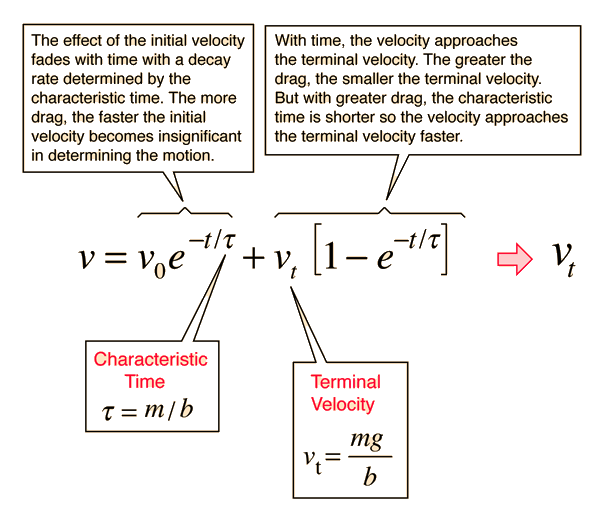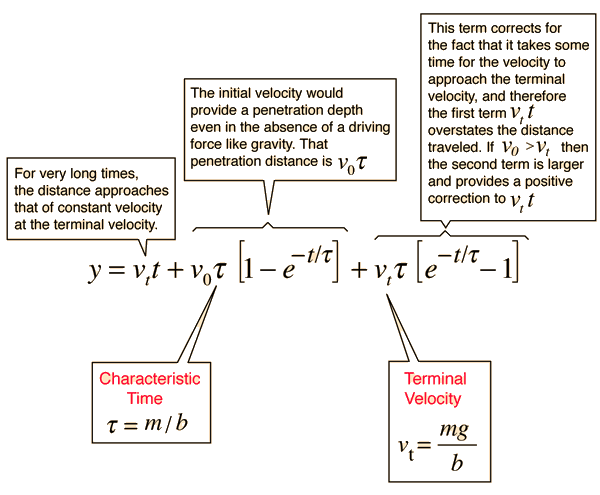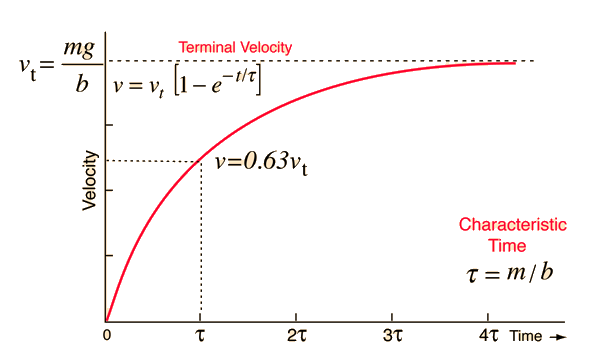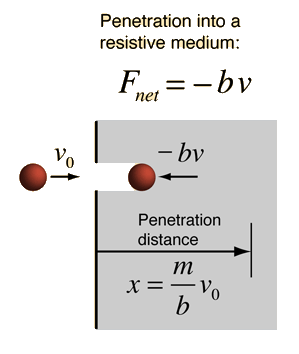# Velocity with Linear Drag

For an object that is acted upon by its weight, mg, and subject to a drag force proportional to its velocity -bv, the general form for the velocity is given by the expression below. For an object in a fluid, you must use an effective force mg' to account for the buoyancy of the fluid. For viscous drag, the drag coefficient b may be expressed in terms of the viscosity of the fluid. This velocity expression may then be integrated to obtain an expression for the distance.Motion equations Graph of velocity Distance details
Index

Fluid friction

 HyperPhysics***** Mechanics ***** Fluids R Nave
Go Back

# Distance with Linear Drag

For an object that is acted upon by its weight, mg, and subject to a drag force proportional to its velocity -bv, the general form for the distance traveled is given by the expression below. For an object in a fluid, you must use an effective force mg' to account for the buoyancy of the fluid. For viscous drag, the drag coefficient b may be expressed in terms of the viscosity of the fluid. You can take the derivative of this distance expression to obtain an expression for the velocity.Motion equations Velocity details
Index

Fluid friction

 HyperPhysics***** Mechanics ***** Fluids R Nave
Go Back

# Velocity Graph, Linear DragThis behavior of velocity as a function of time would be expected for an object released from rest in a viscous medium where the resistance was proportional to velocity. The g value would be adjusted if there was significant buoyancy, and usually is then written g'.

 Motion equations Velocity details
Index

Fluid friction

 HyperPhysics***** Mechanics ***** Fluids R Nave
Go Back

# Penetration of a Viscous Medium

### Sinking objectIndex

Fluid friction

 HyperPhysics***** Mechanics ***** Fluids R Nave
Go Back